Question

# Pole-Zero Diagrams

The following pole-zero diagrams represent the transfer function, H(s) of four different systems. For each system find

a.  the damping factor (or damping ratio)

b.the natural frequency, con

c.  the damped frequency,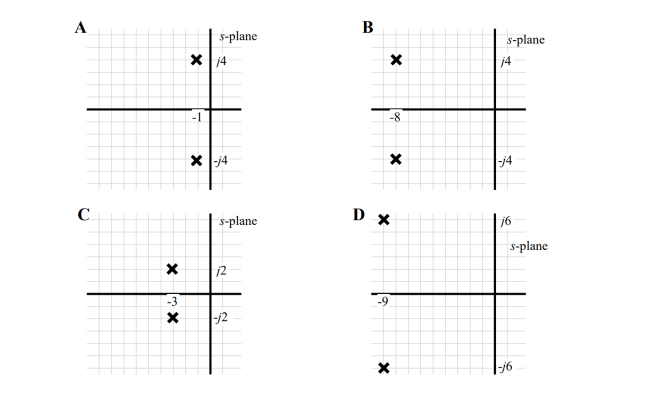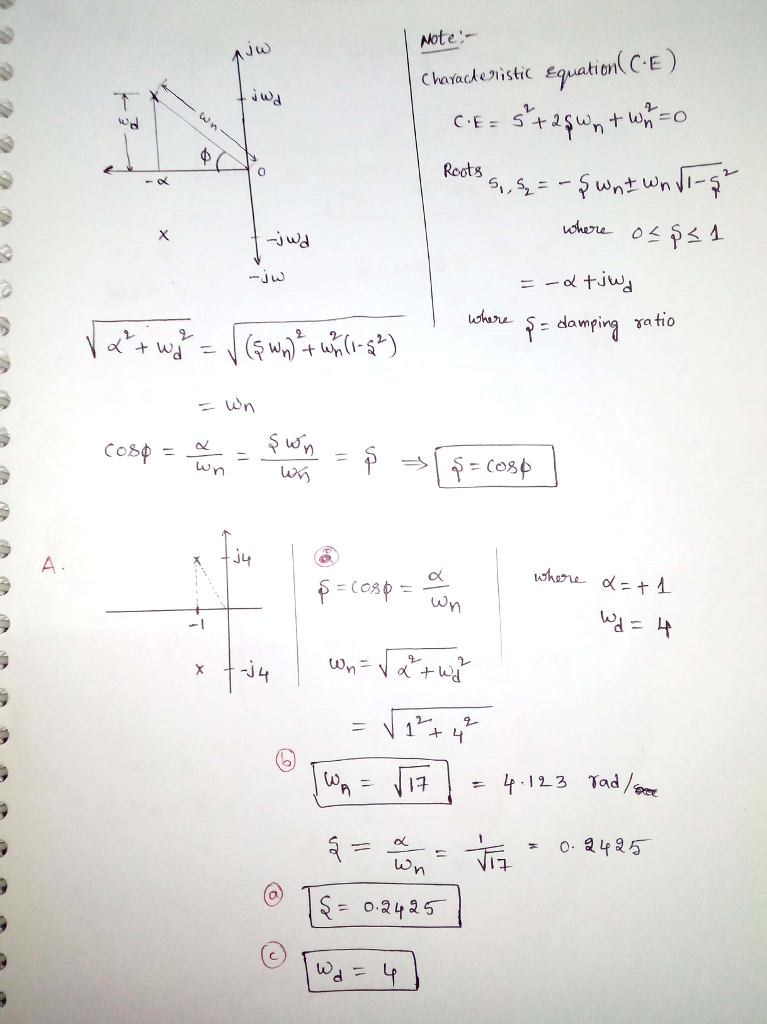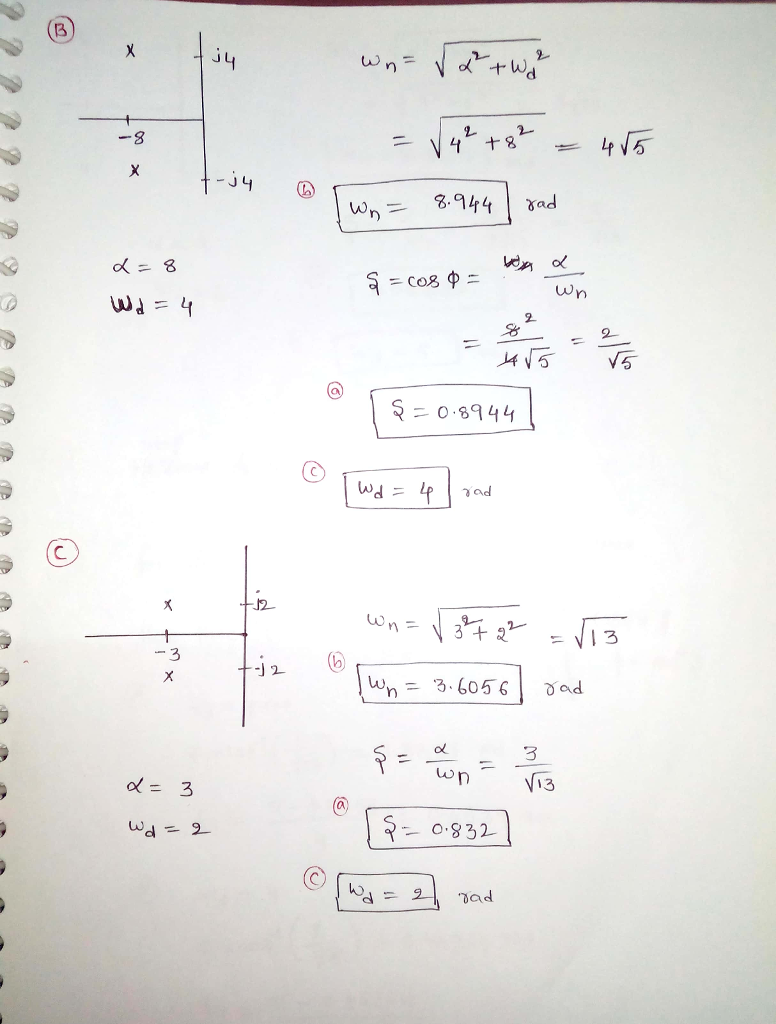> Thanks You so much

Harry Smi Thu, Sep 30, 2021 9:09 AM

#### Earn Coins

Coins can be redeemed for fabulous gifts.

Similar Homework Help Questions
• ### A 2nd order dynamic system has a damping ratio, ζ = 0.5 andnatural frequency, ωn...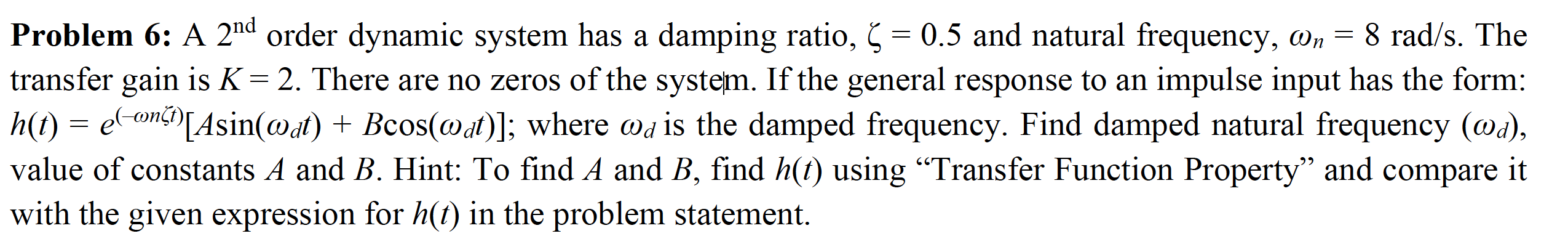A 2nd order dynamic system has a damping ratio, ζ = 0.5 and natural frequency, ωn = 8 rad/s. The transfer gain is K = 2. There are no zeros of the system. If the general response to an impulse input has the form:h(t) =e(–ωnζt)[Asin(ωdt) + Bcos(ωdt)]; whereωd is the damped frequency. Find damped natural frequency (ωd), value of constants A and B. Hint: To find A and B, find h(t) using “Transfer Function Property” and compare it with the given expression...

• ### Design a PD controller for mass-spring systems by the Root-Locus Method Mass 2.6Kg Spring stiffness 200N/m Zero Damp...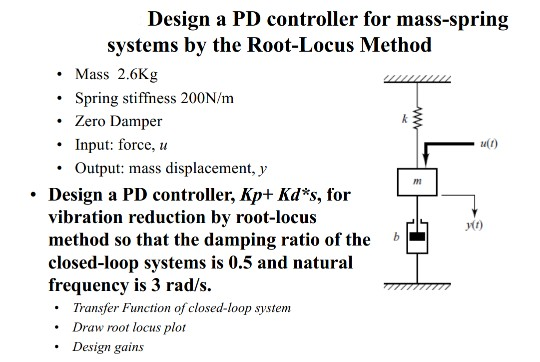Design a PD controller for mass-spring systems by the Root-Locus Method Mass 2.6Kg Spring stiffness 200N/m Zero Damper Input: force Output: mass displacement, y Design a PD controller, Kp+ Kd*s, for vibration reduction by root-locus method so that the damping ratio of the closed-loop systems is 0.5 and natural frequency is 3 rad/s Transfer Function of closed-loop system Draw root locus plot Design gains ww Design a PD controller for mass-spring systems by the Root-Locus Method Mass 2.6Kg Spring stiffness...

• ### Signals and Systems 2. The pole-zero diagram below has 2 zeros at the origin and 2...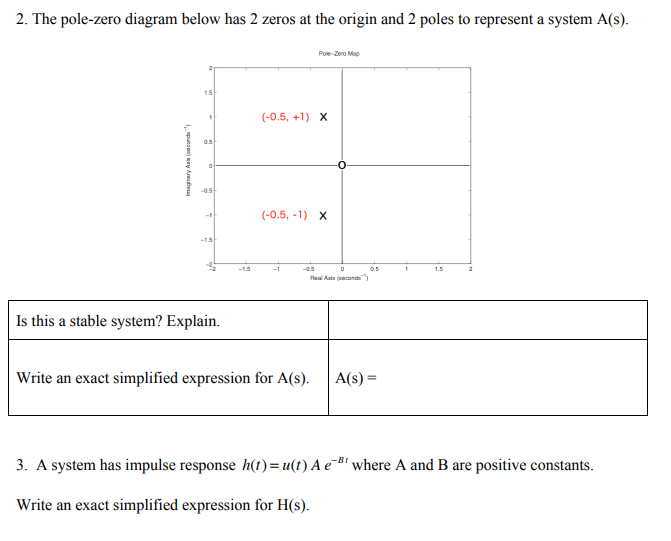Signals and Systems 2. The pole-zero diagram below has 2 zeros at the origin and 2 poles to represent a system A(s). Pole-Zero Map (-0.5, +1) X d Imaginary Au (-0.5, -1) X RealAxis con Is this a stable system? Explain. Write an exact simplified expression for A(s). A(s) = 3. A system has impulse response h(t)= u(t) A e' where A and B are positive constants. Write an exact simplified expression for H(S).

• ### Automatic Control IV Question 4 The transfer function of a servo system has the transfer function...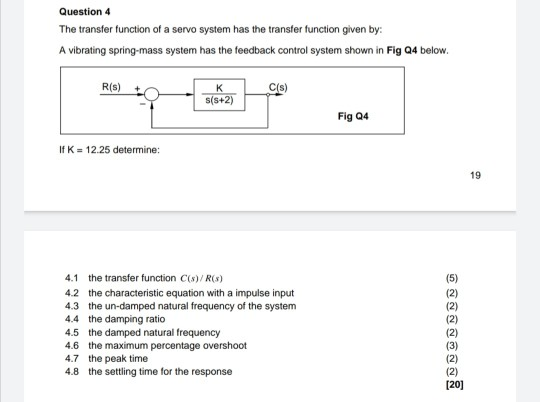Automatic Control IV Question 4 The transfer function of a servo system has the transfer function given by: A vibrating spring-mass system has the feedback control system shown in Fig Q4 below. R(S) - _K s(s+2) Fig 24 If K = 12.25 determine: 4.1 the transfer function C(s)/R(3) 4.3 the un-damped natural frequency of the system 4.4 the damping ratio 4.5 the damped natural frequency 4.6 the maximum percentage overshoot 4.7 the peak time 4.8 the settling time for the...

• ### For the circuit obtain the transfer function E (0) / E (i) and determine the values ​​of the resi...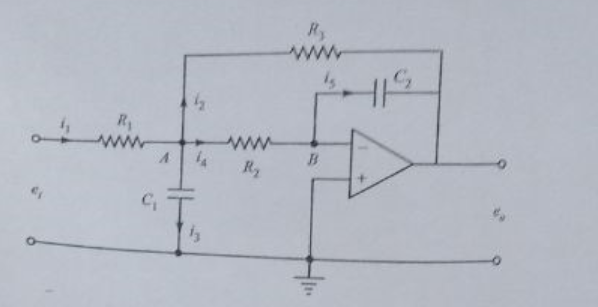For the circuit obtain the transfer function E (0) / E (i) and determine the values ​​of the resistors and capacitors such that the system has a relative damping factor of 0.7 and natural damped frequency of 5rad / sec Draw the entrance and exit in front of the entry and exit time for a unit ramp Find the times ts, tp, tr (for a criterion of 2%) and overshoot of 4% relative damping factor of 0.7 natural damped frequency...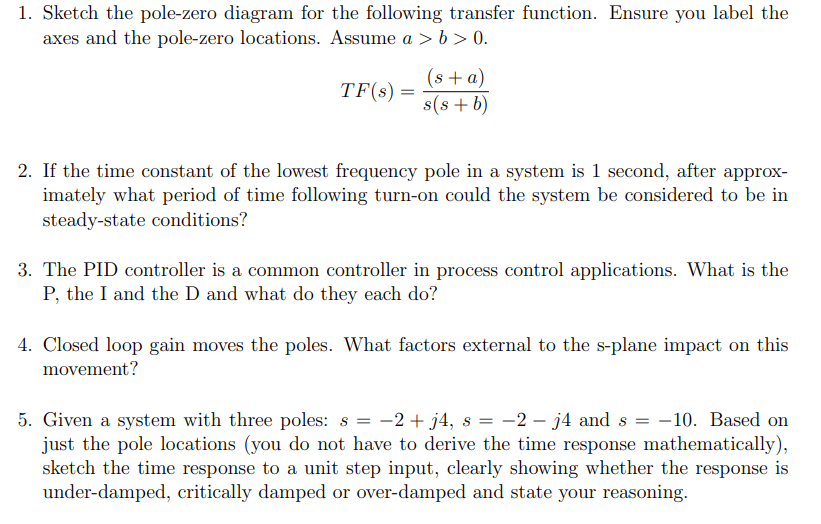please help answer all the question and dont skip. Thank you! 1. Sketch the pole-zero diagram for the following transfer function. Ensure you label the axes and the pole-zero locations. Assume a > b > 0. TF(s) = (s + a) s(s+b) 2. If the time constant of the lowest frequency pole in a system is 1 second, after approx- imately what period of time following turn-on could the system be considered to be in steady-state conditions? 3. The PID...

• ### 5. For each of the following, determine if the system is underdamped, undamped, critically damped or overdamped ad sket...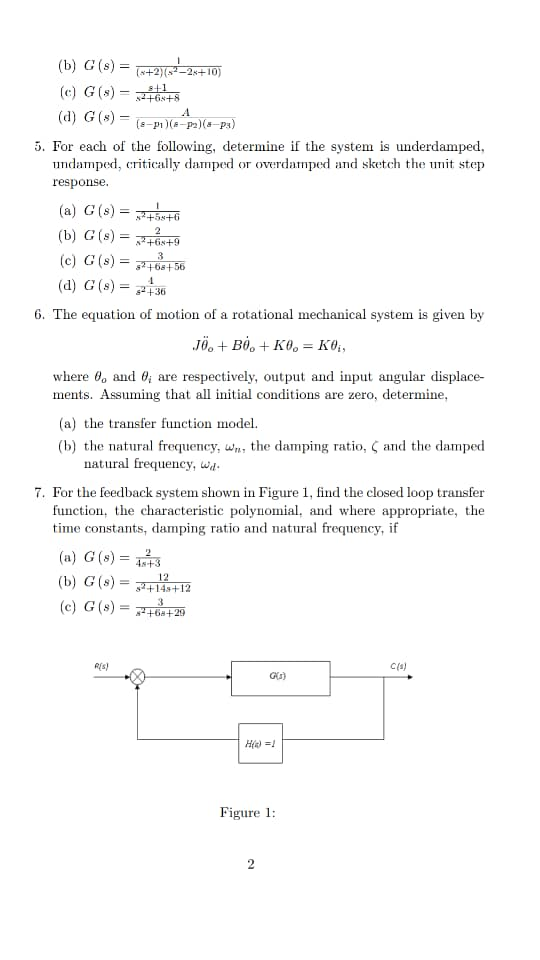5. For each of the following, determine if the system is underdamped, undamped, critically damped or overdamped ad sketch the it step response (a) G (s) = (c) G(s)-t 2+68+ (d) G (s) = 36 6. The equation of motion of a rotational mechanical system is given by where θ° and θί are respectively, output and input angular displace- ments. Assuming that all initial conditions are zero, determine (a) the transfer function model. (b) the natural frequency, w natural frequency,...

• ### 53. For each of the systems with the pole-zero diagrams in Figure E.53 find the discrete-time...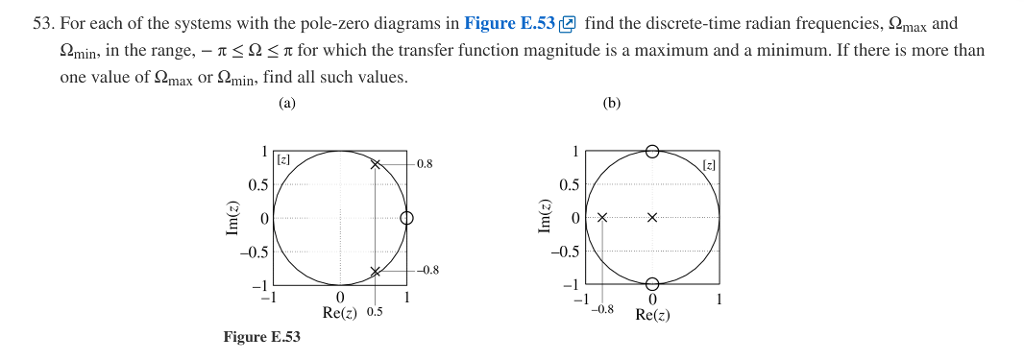53. For each of the systems with the pole-zero diagrams in Figure E.53 find the discrete-time radian frequencies, Ω max and Ωmin, in the range,-π Ω π for which the transfer function magnitude is a maximum and a minimum. If there is more than one value of S2max or S2,min, find all such values [zl 0.8 z] 0.5 0.5 -0.5 -0.5 0.8 -1 -1 0 Re(z) 0.5 0 08 Re() Figure E.53

• ### Slep Response 10 0. 0 1.5 2 Tme (seconds) 05 2.5 35 Question three The figure...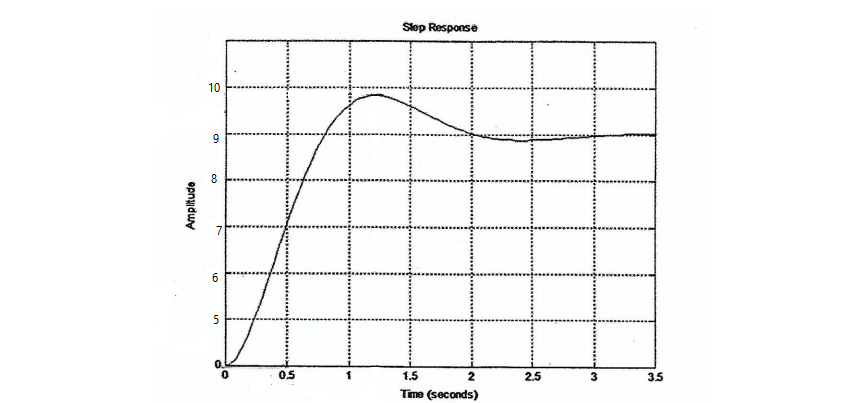Slep Response 10 0. 0 1.5 2 Tme (seconds) 05 2.5 35 Question three The figure below shows a unit step response of a second order system. Prom the graph of response find 1- The rise timet, 2- The peak timet 3- The maximum overshoot M 4 The damped natural frequency w S. The transfer function Hence find the damping ratio ζ and the natural frequency the system Find also the transfer function of

• ### The figure shows the pole diagrams and frequency responses of four continuous-time LTI systems. B...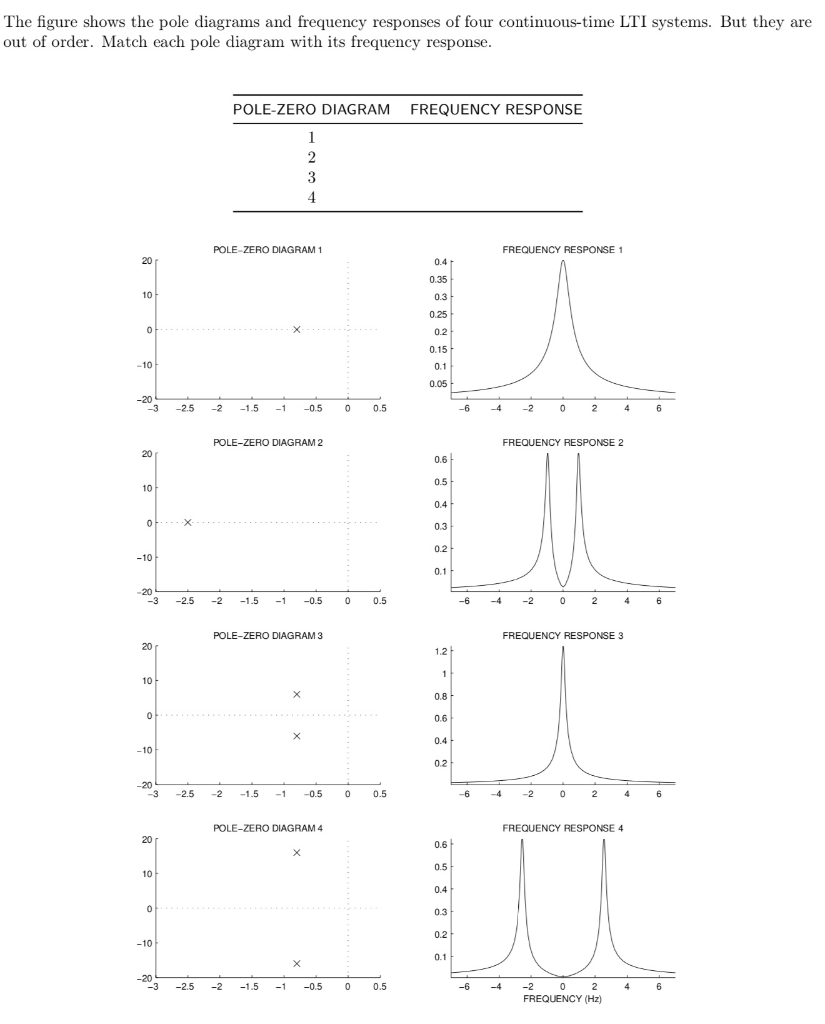signals and systems The figure shows the pole diagrams and frequency responses of four continuous-time LTI systems. But they are out of order. Match each pole diagram with its frequency response POLE-ZERO DIAGRAM FREQUENCY RESPONSE POLE-ZERO DIAGRAM1 FREQUENCY RESPONSE1 0.4 0.35 0.3 0.25 0.15 0.1 0.05 3 2.5 -2 1.5.5 00.5 -6 42 POLE-ZERO DIAGRAM 2 FREQUENCY RESPONSE 2 0.6 0.5 0.4 0.3 10 -10 0.1 -3 -2.5 -2 1.5-0.5 0 0.5 -6 -42 POLE-ZERO DIAGRAM 3 FREQUENCY RESPONSE 3...